Courses

# Influence Lines for Beams (Part - 3) Civil Engineering (CE) Notes | EduRev

## Civil Engineering (CE) : Influence Lines for Beams (Part - 3) Civil Engineering (CE) Notes | EduRev

The document Influence Lines for Beams (Part - 3) Civil Engineering (CE) Notes | EduRev is a part of the Civil Engineering (CE) Course Structural Analysis.
All you need of Civil Engineering (CE) at this link: Civil Engineering (CE)

Numerical Example

Compute maximum end shear for the given beam loaded with moving loads as shown in Figure 39.8.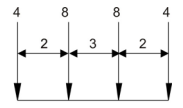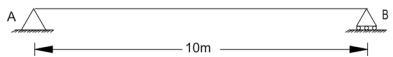When first load of 4 kN crosses support A and second load 8 kN is approaching support A, then change in shear can be given by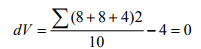When second load of 8 kN crosses support A and third load 8 kN is approaching support A, then change in shear can be given by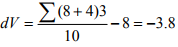Hence, as discussed earlier, the second load 8 kN has to be placed on support A to find out maximum end shear (refer Figure 39.9).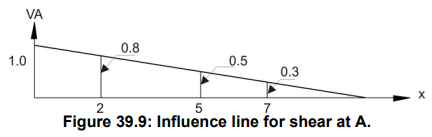VA = 4 × 1 + 8 × 0.8 + 8 × 0.5 + 4 × 0.3 = 15.6kN

Maximum shear at a section in a beam supporting a series of moving concentrated loads

In this section we will discuss about the case, when a series of concentrated loads are moving on beam and we are interested to find maximum shear at a section. Let us assume that series of loads are moving from right end to left end as shown in Figure. 39.10.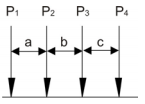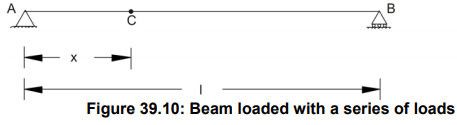The influence line for shear at the section is shown in Figure 39.11.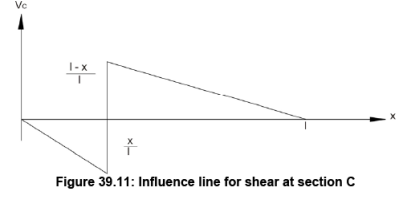Monitor the sign of change in shear at the section from positive to negative and apply the concept discussed in earlier section. Following numerical example explains the same.

Numerical Example

The beam is loaded with concentrated loads, which are moving from right to left as shown in Figure 39.12. Compute the maximum shear at the section C.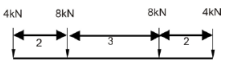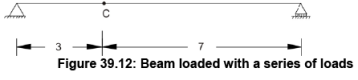The influence line at section C is shown in following Figure 39.13.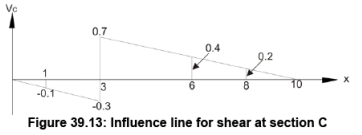When first load 4kN crosses section C and second load approaches section C then change in shear at a section can be given by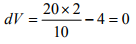When second load 8 kN crosses section C and third load approaches section C then change in shear at section can be given by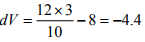Hence place the second concentrated load at the section and computed shear at a section is given by

VC = 0.1 × 4 + 0.7 × 8 + 0.4 × 8 + 0.2 × 4 = 9.2kN

Maximum Moment at a section in a beam supporting a series of moving concentrated loads

The approach that we discussed earlier can be applied in the present context also to determine the maximum positive moment for the beam supporting a series of moving concentrated loads. The change in moment for a load P1 that moves from position x1 to x2 over a beam can be obtained by multiplying P1 by the change in ordinate of the influence line i.e. (y2 – y1). Let us assume the slope of the influence line (Figure 39.14) is S, then (y2 – y1) = S (x2 – x1).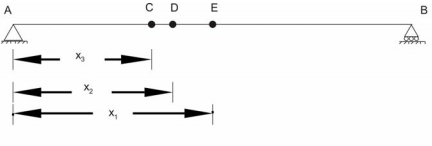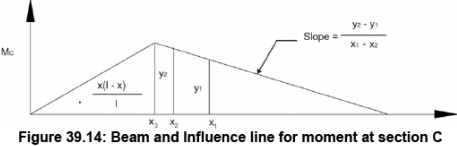Hence change in moment can be given by

dM = P1S(x2 - x1)

Let us consider the numerical example for better understanding of the developed concept.

Numerical Example

The beam is loaded with concentrated loads, which are moving from right to left as shown in Figure 39.15. Compute the maximum moment at the section C.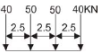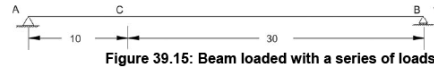The influence line for moment at C is shown in Figure 39.16.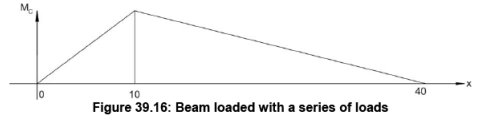If we place each of the four-concentrated loads at the peak of influence line, then we can get the largest influence from each force. All the four cases are shown in Figures 39.17-20.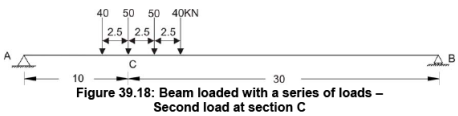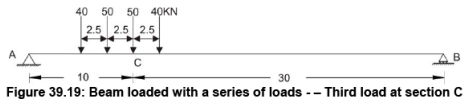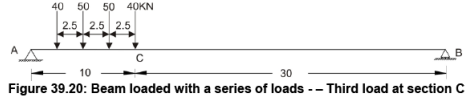As shown in Figure 39.17, when the first load crosses the section C, it is observed that the slope is downward (7.5/10). For other loads, the slope is upward (7.5/(40-10)). When the first load 40 kN crosses the section and second load 50 kN is approaching section (Figure 39.17) then change in moment is given by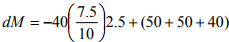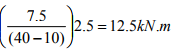When the second load 50 kN crosses the section and third load 50 kN is approaching section (Figure 39.18) then change in moment is given by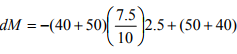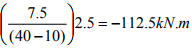At this stage, we find negative change in moment; hence place second load at the section and maximum moment (refer Figure 39.21) will be given by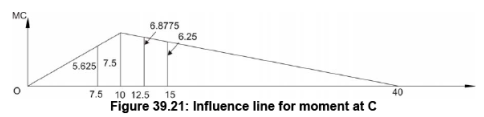Mc = 40(5.625)+ 50(7.5)+ 50(6.8775) + 40(6.25) = 1193.87kNm

Offer running on EduRev: Apply code STAYHOME200 to get INR 200 off on our premium plan EduRev Infinity!

## Structural Analysis

28 videos|114 docs|27 tests

,

,

,

,

,

,

,

,

,

,

,

,

,

,

,

,

,

,

,

,

,

;